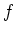Previous: 8.2 Discrete Wigner Transform Up: 8. Finite Difference Wigner Next: 8.3.1 Continuity Equation

# 8.3 Conservation of Mass

There are two basic theories to describe nonequilibrium phenomena in mechanics: kinetic theory from a microscopic level and continuum theory from the macroscopic level. For the NANOTCAD project we adopted a first principles approach using a microscopic formulation. This leads us to the Wigner (quantum case) and to the Boltzmann equation (semiclassical case) which describe transport by a distribution functionin six-dimensional phase space.

For many TCAD purposes this kinetic description contains more information than we really need and it is numerically not tractable. The link to the continuum formulation is made by reducing the distribution to its first few velocity moments. Of particular interest are the moments associated with the mass, momentum and kinetic energy. The equations for these macroscopic variables are called conservation equations, because they describe quantities which are conserved due to physical symmetries (Nöther's theorem).

All the moment equations are derived from the Wigner equation and are implicitly fulfilled by the distribution function. When discretizing the Wigner equation, one has to take great care that the discrete Wigner equation also leads to a reasonable discrete conservation law and to correct continuum physics. In this section we study the conservation of mass and the constraints this imposes on the mesh.

SubsectionsPrevious: 8.2 Discrete Wigner Transform Up: 8. Finite Difference Wigner Next: 8.3.1 Continuity Equation